### IMO Shortlist 2016 problem G1

Kvaliteta:
Avg: 0,0
Težina:
Avg: 6,0

Triangle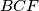$BCF$ has a right angle at$B$. Let$A$ be the point on line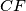$CF$ such that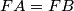$FA=FB$ and$F$ lies between$A$ and$C$. Point$D$ is chosen so that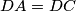$DA=DC$ and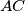$AC$ is the bisector of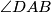$\angle{DAB}$. Point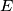$E$ is chosen so that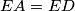$EA=ED$ and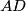$AD$ is the bisector of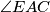$\angle{EAC}$. Let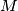$M$ be the midpoint of$CF$. Let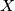$X$ be the point such that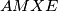$AMXE$ is a parallelogram. Prove that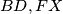$BD,FX$ and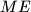$ME$ are concurrent.

Izvor: https://www.imo-official.org/problems/IMO2016SL.pdf